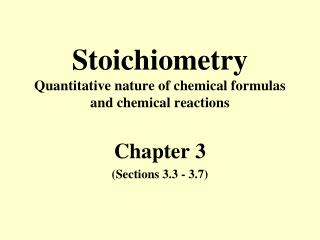# Stoichiometry Quantitative nature of chemical formulas and chemical reactions - PowerPoint PPT PresentationDownload PresentationStoichiometry Quantitative nature of chemical formulas and chemical reactions

Stoichiometry Quantitative nature of chemical formulas and chemical reactions
Download Presentation## Stoichiometry Quantitative nature of chemical formulas and chemical reactions

- - - - - - - - - - - - - - - - - - - - - - - - - - - E N D - - - - - - - - - - - - - - - - - - - - - - - - - - -
##### Presentation Transcript

1. StoichiometryQuantitative nature of chemical formulas and chemical reactions Chapter 3 (Sections 3.3 - 3.7)

2. Atomic Mass Scale • Atomic mass units (amu) are convenient units to use when dealing with extremely small masses of individual atoms • 1 amu = 1.66054 x 10-24 g • 1 g = 6.02214 x 1023 amu • By definition, the mass of C-12 is exactly 12 amu

3. Average Atomic Mass(Atomic Weight) • We average the masses of isotopes using their masses and relative abundances to give the average atomic mass of an element. •  Naturally occurring C consists of 98.892% C-12 (12.00 amu) and 1.108% C-13 (13.00335 amu) •  The average mass of C is • (0.98892)(12.00 amu) + (0.01108)(13.00335) = 12.01 amu • Atomic weights are listed on the periodic table

4. Calculating % Abundance • Chlorine is made up of two isotopes, Cl-35 atomic mass = 34.969 amu and Cl- 37 atomic mass = 36.966 amu. Given chlorine’s atomic weight of 35.453, what is the % abundance of each isotope? 34.969(x) + (36.966) (1-x) = 35.453 34.969x + 36.966 – 36.966x = 35.453 x = .7576 75.76% Cl-35 and 24.24% Cl-37

5. Formula & Molecular Weights • Formula Weight is the sum of atomic weights for the atoms present in the chemical formula • Molecular Weight is the sum of atomic weights of the atoms in a molecule as shown in the molecular formula • Sample Exercise 3.5 page 80

6. Percentage Composition from Formulas • Obtained by dividing the mass contributed by each element (number of atoms times atomic weight) by the formula weight of the compound and multiplying by 100 • Sample Exercise 3.6 page 80

7. The Mass Spectrometer page 81 • Mass spectrometers are pieces of equipment designed to measure atomic and molecular masses accurately. • The sample is converted to positive ions by collisions with a stream of high-energy electrons upon entering the spectrometer. • The charged sample is accelerated using an applied voltage. • The ions are then passed into an evacuated tube through a magnetic field. • The magnetic field causes the ions to be deflected by different amounts depending on their mass – more mass, less deflection. • The ions are then detected.

8. The Mole • The mole is a convenient measure of chemical quantities.  • 1 mole of something is 6.0221421 x 1023 of that thing. • This number is called Avagadro’s number. • Thus 1 mole of carbon atoms = 6.0221421 x 1023 carbon atoms

9. Molar Mass • mass in grams of 1 mole of a substance •  expressed in units of g/mol • formula weights are numerically equal to the molar mass • Sample Exercise 3.8 page 84

10. Interconverting Masses, Moles, & Number of Particles • To convert between grams and moles, we use the molar mass • To convert between moles and particles (atoms, molecules, or ions) we use Avogadro’s number • Sample Exercises 3.7, 3.9, 3.10, 3.11 pages 82-86

11. Empirical Formula • Gives the relative number of atoms of each element in the substance • Can be calculated from mass percent data • Sample Exercise 3.12 page 87

12. Molecular Formula • Actual number of atoms of each element in one molecule of the substance • Whole number multiple of empirical formula • Sample Exercise 3.13 page 88

13. Combustion Analysis • A sample containing C, H, and O is combusted in excess oxygen to produce CO2 & H2O • Can be used to determine empirical formula or percent composition

14. Quantitative Information from Balanced Equations • Coefficients can be interpreted as the relative numbers of molecules or formula units in the reaction as well as the relative number of moles • See Figure 3.14 page 92 • Sample Exercises 3.14, 13.15 pages 92 & 93

15. Limiting Reactant(s) • The reactant(s) that is completely consumed in a reaction • Limits or determines the amount of product that will be formed • The other reactant(s) that is left over is called the excess reactant • Sample Exercise 3.16 and 3.17 pages 95-96 • How much of the excess reactant is left over?

16. Percent Yield (actual yield / theoretical yield) X 100 • Actual is the amount of product recovered in the lab • Theoretical is the amount predicted from stoichiometry • Sample Exercise 3.18 page 97